\$B:#>,\$N0lGU(B

\$BKhF|!":#>,\$N0lGU\$r>e\$2\$F\$\$\$^\$9!#(B
\$B:#>,0{\$`\$b\$N\$K:\$\$C\$?\$i!";29M\$K\$7\$F\$_\$F\$/\$@\$5\$\$!#(B

2020\$BG/(B10\$B7n(B

 1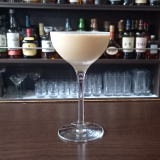(I<^*HWY%E/B(B 2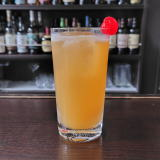(I@^2TS]D^%M/D^(B 3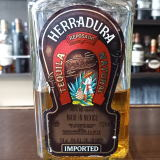(I4WD^)0W\$B!!(IZN_;D^(B 4 \$B5Y\$_(B 5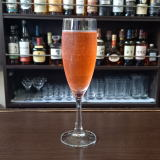(ILWP]:^(B 6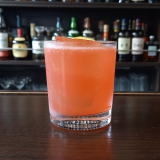(IA,J_W(B 7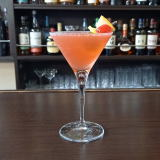(I1:0@^]=(B 8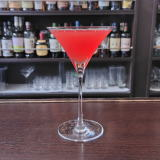(IWQ0Y(B 9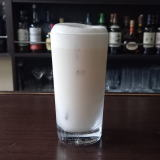(IL^W]C^0%PY8%J_]A(B 10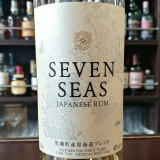(I>L^]%<0=^(B 11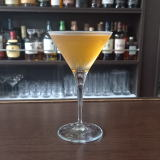(ILW]<=%1YJ^0D(B 12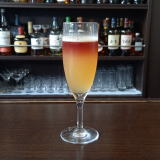(IF-0V08%;\0(B 13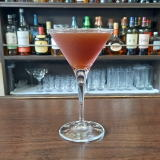(I2]8^X/<-%L^W/8?0](B 14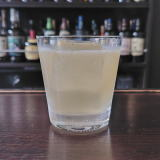(I1D^PWY(B 15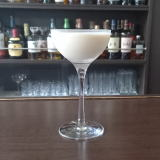(IQ0P](B 16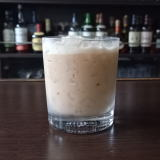(I=:/A%50Z(B 17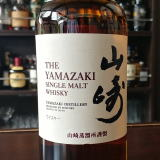\$B;3:j(B 18 \$B5Y\$_(B 19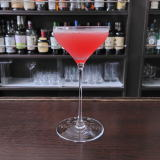(I8[3(B 20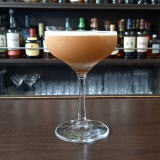(IK^X0%JPYD](B 21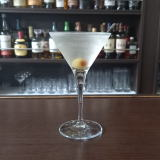(ID^W2%OC(0F(B 22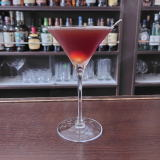(IK_]N_]%68CY(B 23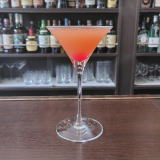(ID\2W2D%?^0](B 24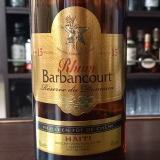(IJ^YJ^]80Y(B15\$BG/(B 25 \$B5Y\$_(B 26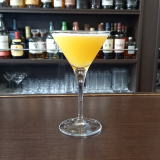(ILW%L0L_(B 27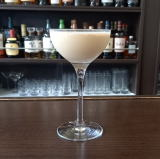(I1Z7;]@^0=^%L^W;^0(B 28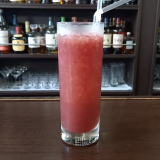(IK^<./L_(B 29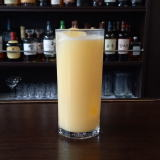(ILYL(0%@^/8(B 30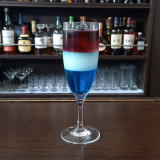(IDX:[0Y(B 31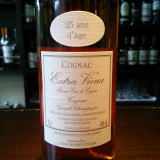(IN_0Y%<^[0(B25\$BG/(B